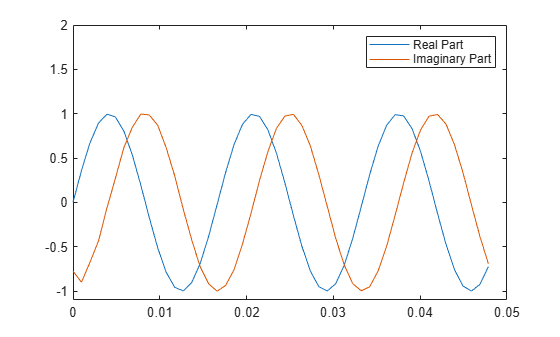# Hilbert Transform

The Hilbert transform facilitates the formation of the analytic signal. The analytic signal is useful in the area of communications, particularly in bandpass signal processing. The toolbox function `hilbert` computes the Hilbert transform for a real input sequence `x` and returns a complex result of the same length, `y = hilbert(x)`, where the real part of `y` is the original real data and the imaginary part is the actual Hilbert transform. `y` is sometimes called the analytic signal, in reference to the continuous-time analytic signal. A key property of the discrete-time analytic signal is that its Z-transform is 0 on the lower half of the unit circle. Many applications of the analytic signal are related to this property; for example, the analytic signal is useful in avoiding aliasing effects for bandpass sampling operations. The magnitude of the analytic signal is the complex envelope of the original signal.

The Hilbert transform is related to the actual data by a 90-degree phase shift; sines become cosines and vice versa. To plot a portion of data and its Hilbert transform, use

```t = 0:1/1024:1; x = sin(2*pi*60*t); y = hilbert(x); plot(t(1:50),real(y(1:50))) hold on plot(t(1:50),imag(y(1:50))) hold off axis([0 0.05 -1.1 2]) legend('Real Part','Imaginary Part')```The analytic signal is useful in calculating instantaneous attributes of a time series, the attributes of the series at any point in time. The procedure requires that the signal be monocomponent.